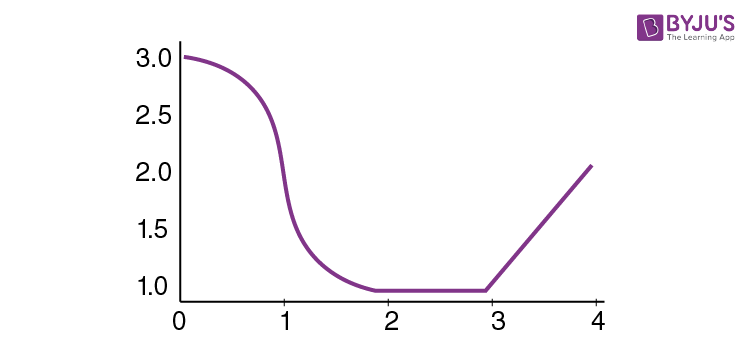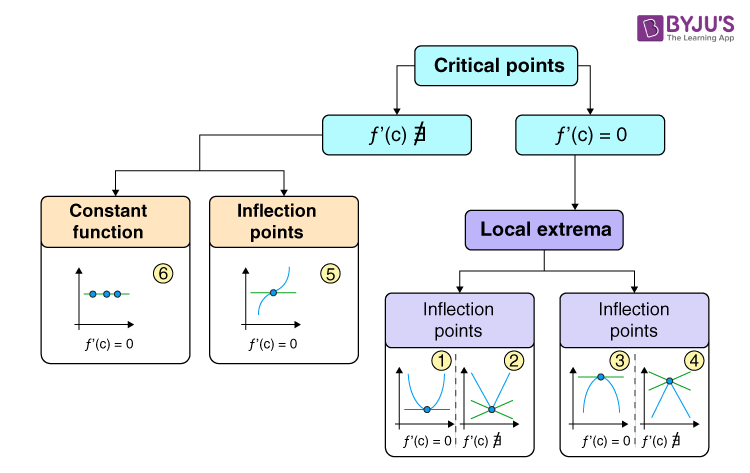Checkout JEE MAINS 2022 Question Paper Analysis : Checkout JEE MAINS 2022 Question Paper Analysis :

# Critical Points Solved Examples

A point c in the domain of a function f(x) is called a critical point of f(x), if f ‘(c) = 0 or f ‘(c) does not exist. This article explains the critical points along with solved examples.

A function f which is continuous with x in its domain contains a critical point at point x if the following conditions hold good.

• f ’(x) = 0
• f ’(x) is undefined.

A point of a differentiable function f at which the derivative is zero can be termed as a critical point.

The types of critical points are as follows:

• A critical point is a local maximum if the function changes from increasing to decreasing at that point, whereas it is called a local minimum if the function changes from decreasing to increasing at that point.
• A critical point is an inflexion point if the concavity of the function changes at that point.
• If a critical point is neither of the above, then it signifies a vertical tangent in the graph of a function.This function has critical points at x = 1 and x = 3.

Classification of critical points is explained below:## Solved Problems On Critical Points

Example 1: Find the critical points of the function f (x) = x2 lnx.

Solution:

Take the derivative using the product rule:

f ′ (x) = (x2 lnx)′ = 2x * ln x + x2 * [1 / x] = 2x ln x + x = x (2 ln x + 1).

Determine the points where the derivative is zero:

f′(c) = 0, ⇒ c (2 ln c + 1) = 0.

The first root c1 = 0 is not a critical point because the function is defined only for x > 0.

Consider the second root:

2 ln c + 1 = 0, ⇒ ln c =−1 / 2,

⇒ c2 = e −1/2 = 1 / √e.

Hence, c2 = 1 / √e is a critical point of the given function.

Example 2: Local maximum and local minimum values of the function (x − 1) (x + 2)2 are

Solution:

f′(x) = 2 (x − 1) (x + 2) + (x + 2)2 = 3x2 + 6x

f′(x) = 0

x = 0, −2

f (−2) = (−2 −1) (−2 + 2)2 = 0 (Maximum value) and

f (0) = (0 − 1) (0 + 2)2 = −4 (Minimum value).

Example 3: Whether the given function x * √[1 − x2], (x > 0) has local maxima or minima?

Solution:

Let f (x) = x * √[1 − x2]

f′(x) = [1 − 2x2] / √[1 − x2] = 0

⇒ x = ± 1 / √2

But as x > 0, we have x = 1 / √2

Now, again f′′(x) = {(√[1 − x2] (−4x)) − ((1 − 2x2) * (− x / √[1 − x2])} / [1 − x2] = [2x3 − 3x] / (1 − x2)3/2

⇒ f′′(1 / √2) = −ve.

Then, f (x) is maximum at x = 1 / √2.

Example 4: The area of a rectangle will be maximum for the given perimeter when a rectangle is a ________.

Solution:

We know that the perimeter of a rectangle S = 2 (x + y), where x and y are adjacent sides.

y = [S − 2x] / 2.

Now the area of a rectangle,

A = xy = [x / 2] * (S − 2x) = [1 / 2] * (Sx − 2x2)

Differentiating w.r.t. x of A, we get

dA / dx = 1 / 2 (S − 4x) = 0

∴x = S / 4 and y = S / 4

Again d2A / dx2 = −ve

Hence, the area of the rectangle will be maximum when the rectangle is a square.

Example 5: If f (x) = 2x3 − 3x2 − 12x + 5 and x ∈ [−2, 4], then the maximum value of the function is at what value of x?

Solution:

f′(x) = 6x2 − 6x − 12

f′(x) = 0

⇒ (x − 2) (x + 1) = 0

⇒ x = −1, 2

Here f (4) = 128 − 48 − 48 + 5 = 37

f (−1) = −2 − 3 + 12 + 5 = 12

f (2) = 16 − 12 − 24 + 5 = −15

f (−2) = −16 − 12 + 24 + 5 = 1

Therefore, the maximum value of function is 37 at x = 4.

Example 6: The function sinx (1 + cosx) at x = π / 3, is ____________.

Solution:

Let f (x) = sinx (1 + cosx)

f′(x) = cos2x + cosx and f′′(x) =−2sin2x − sinx = −(2sin2x + sinx)

For maximum or minimum value of f (x), f′(x) = 0

cos2x + cosx = 0

cos x = −cos2x

cosx = cos (π ± 2x)

∴ x = π ± 2x or x = π / 3, −π

Now f′′(π / 3) = −2sin 2π / 3 − sin π / 3 = [−2] * [(√3) / 2] − √3 / 2 = −3√3 / 2 =−ve

Hence, f (x)is maximum at x = π / 3.

Example 7: If x + y = 10, then the maximum value of xy is __________.

Solution:

x + y =10

∴ y = 10 − x ……..(i)

Now f (x) = xy = x (10 − x) = 10x − x2

∴ f′(x) = 10 − 2x

For maximum value of f (x), f′ (x) = 0

∴ x = 5 and y = 5

So the maximum value of xy = 5 × 5 = 25.

Example 8: The point (0, 5) is closest to the curve

$$\begin{array}{l}{{x}^{2}}=2y\end{array}$$
is

Solution:

Let a point on the curve by (h, k).

Then

$$\begin{array}{l}{{h}^{2}}=2k \rightarrow (i)\end{array}$$

Distance = D =

$$\begin{array}{l}\sqrt{{{h}^{2}}+{{(k-5)}^{2}}}\end{array}$$

By (i);

$$\begin{array}{l}D=\sqrt{2k+{{(k-5)}^{2}}}\\ \frac{dD}{dk}=\frac{1}{2\sqrt{2k+{{(k-5)}^{2}}}}\times 2(k-5)+2=0 \ x\in (-1,\infty )\end{array}$$

So, at k = 4 function D must be minimum.

Then point will be

$$\begin{array}{l}(\pm \,2\sqrt{2},\,4).\end{array}$$

Example 9: The minimum value of

$$\begin{array}{l}4{{e}^{2x}}+9{{e}^{-2x}}\end{array}$$
is

A)  11

B) 12

C) 10

D) 14

Solution:

Let

$$\begin{array}{l}f(x)=4{{e}^{2x}}+9{{e}^{-2x}}\\ {f}'(x)=8{{e}^{2x}}-18{{e}^{-2x}}\end{array}$$

Put

$$\begin{array}{l}{f}'(x)=0\Rightarrow 8{{e}^{2x}}-18{{e}^{-2x}}=0\\ {{e}^{2x}}=3/2\Rightarrow x=\log {{(3/2)}^{1/2}}\end{array}$$

Again,

$$\begin{array}{l}{f}”(x)=16{{e}^{2x}}+36{{e}^{-2x}}>0\end{array}$$

Now,

$$\begin{array}{l}f(\log {{(3/2)}^{1/2}})=4{{e}^{2.(\log {{(3/2)}^{1/2}})}}+9{{e}^{-2(\log {{(3/2)}^{1/2}})}} \\ = 4\times \frac{3}{2}+9\times \frac{2}{3} = 6+6=12\end{array}$$

Hence minimum value = 12.

Example 10: The function

$$\begin{array}{l}f(x)={{x}^{-x}},\,(x\,\in \,R)\end{array}$$
attains a maximum value at x =

A) 2

B) 3

C) 1/e

D) 1

Solution:

$$\begin{array}{l}f(x)=y={{x}^{-x}}\\ \log y=-\,x\log x\end{array}$$

Differentiating w.r.t. x,

$$\begin{array}{l}\frac{1}{y}.\frac{dy}{dx}=-\left[ x.\frac{1}{x}+\log x \right]\\ \frac{1}{y}.\frac{dy}{dx}=-[1+\log x]\\ \frac{dy}{dx}=-{{x}^{-x}}[1+\log x] \\ \frac{dy}{dx}={{x}^{-x}}\left[ \log \frac{1}{x}-1 \right]\end{array}$$

Put

$$\begin{array}{l}\frac{dy}{dx}=0\\ {{\log }_{e}}\frac{1}{x}={{\log }_{e}}e\\ \frac{1}{x}=e \Rightarrow x=\frac{1}{e}.\end{array}$$

Example 11: If

$$\begin{array}{l}y=a\,\,\log x+b{{x}^{2}}+x\end{array}$$
has its extremum value at x = 1 and x = 2, then (a, b) =

Solution:

$$\begin{array}{l}\frac{dy}{dx}=\frac{a}{x}+2bx+1\\ {{\left( \frac{dy}{dx} \right)}_{x=1}}=a+2b+1=0\\ a=-2b-1 \text \ and \ {{\left( \frac{dy}{dx} \right)}_{x=2}}=\frac{a}{2}+4b+1=0\\ \frac{-2b-1}{2}+4b+1=0\\ -b+4b+\frac{1}{2}=0\\ 3b=\frac{-1}{2}\\ b=\frac{-1}{6} \text \ and \ a=\frac{1}{3}-1=\frac{-2}{3}\end{array}$$

Example 12: If

$$\begin{array}{l}f(x)=\frac{1}{4{{x}^{2}}+2x+1},\end{array}$$
then find its maximum value.

Solution:

$$\begin{array}{l}f(x)=\frac{1}{4{{x}^{2}}+2x+1}\\ {f}'(x)=\frac{-(8x+2)}{{{(4{{x}^{2}}+2x+1)}^{2}}}\end{array}$$

Put

$$\begin{array}{l}{f}'(x)=0\\ 8x+2=0\\ x=-1/4\\ {f}”\,(x)=\frac{-[{{(4{{x}^{2}}+2x+1)}^{2}}8-(8x+2)\,2\,(4{{x}^{2}}+2x+1)(8x+2)]}{{{(4{{x}^{2}}+2x+1)}^{4}}}\\ {f}”(-1/4)=\text \ -ve \ (point \ of \ maxima) \\ \ f{{(-1/4)}_{\text{max}\text{.}}}\\ =\frac{1}{4\times \frac{1}{16}-2\times \frac{1}{4}+1}=\frac{4}{3}.\end{array}$$